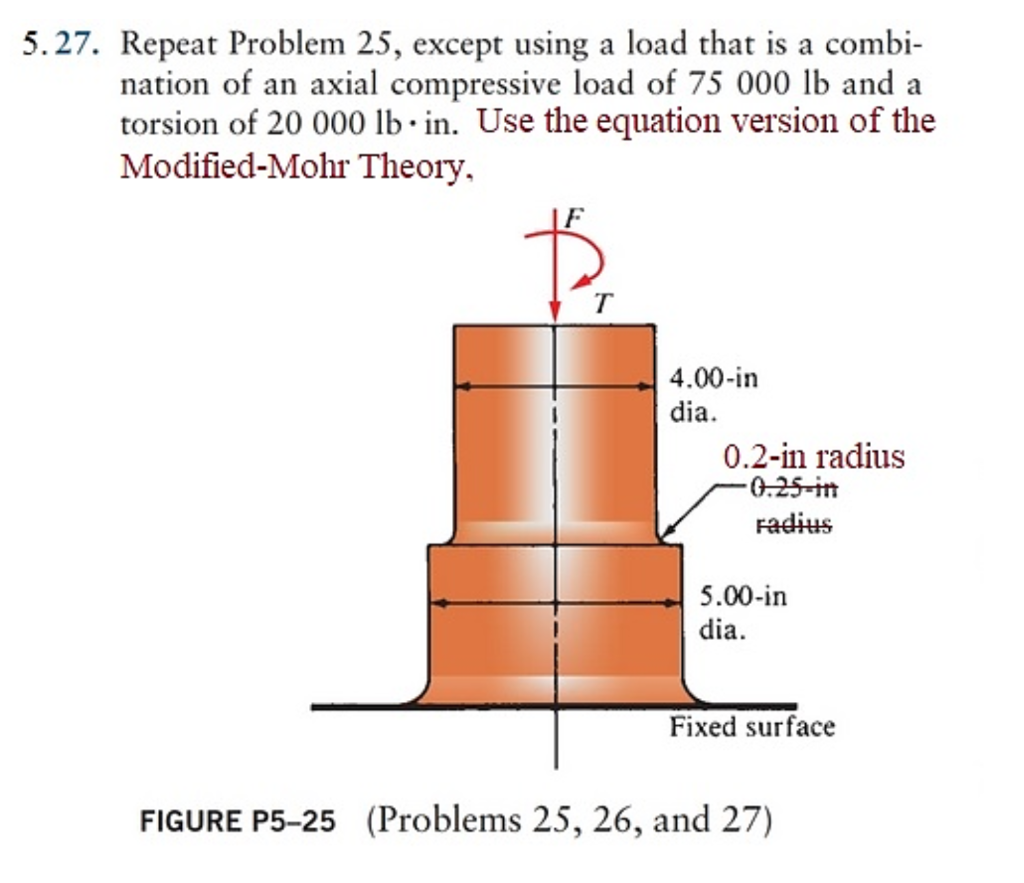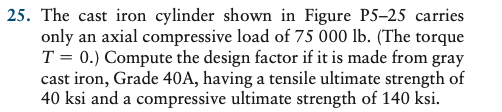# (Solved): 5.27. Repeat Problem 25, Except Using A Load That Is A Combi- Nation Of An Axial Compressive Load Of...5.27. Repeat Problem 25, except using a load that is a combi- nation of an axial compressive load of 75 000 lb and a torsion of 20 000 lb. in. Use the equation version of the Modified-Mohr Theory, 4.00-in dia. 0.2-in radius -0.25-in radius 5.00-in dia. Fixed surface FIGURE P5-25 (Problems 25, 26, and 27) 25. The cast iron cylinder shown in Figure P5-25 carries only an axial compressive load of 75 000 lb. (The torque T = 0.) Compute the design factor if it is made from gray cast iron, Grade 40A, having a tensile ultimate strength of 40 ksi and a compressive ultimate strength of 140 ksi.

We have an Answer from Expert